• 用matlab编写实现最小二乘法多元线性拟合，可以得到最终拟合方程，并画出预测的回归系数直方图
• matlab开发-偏最小二乘法和判别分析法。使用PLS进行判别分析的教程和工具。
• 针对核偏最小二乘法（KPLS）随核函数矩阵维数膨胀而计算量增加的问题，提出分块核偏最小二乘法（BKPLS）。BKPLs根据核函数矩阵对称的性质，将KPLs中的批量算法转变成分块算法，不但减小了对计算机硬件的要求，而且...
• 偏最小二乘法代码PartialLeastSquares_forClassification 用于数据分类的偏最小二乘算法的 Matlab 实现。 这些代码是基于以下论文实现的： Alin, A. (2009) “当对象数量远大于变量数量时 PLS 算法的比较”，统计...
•偏最小二乘法
• 模式识别的一个重要算法：SIMCA（软独立建模聚类分析）你值得拥有
• 偏最小二乘法是一种数学优化技术，它通过最小化误差的平方和找到一组数据的最佳函数匹配。 用最简的方法求得一些绝对不可知的真值，而令误差平方之和为最小。 很多其他的优化问题也可通过最小化能量或最大化熵用最小...
• 针对该问题，提出一种使用核偏最小二乘法来预测故障原因的方法，该方法克服了传统线性回归方法的缺陷，在高维的非线性空间对数据进行分析，同时，该方法也吸收了典型相关分析和主成分分析方法的特点，为分析提供了...无线传感网 核偏最小二乘法
• pretreat.m，pretreat.m ，opls.m ，oscfearn.m loscwold.m ks.m pls.m Idapinv.m plslda.m lecr.m plscv.m, plsidacv.m lplscv.m, plsldacv.m, ecrcv.m plsdcv.m, plsldadcv.m plsmccv.m, plsldamccv.m，mcs.m ...
• 对同色异谱黑进行偏最小二乘分析,提取潜在成分,获得了KMN向量,将其与Lab向量组合成6维向量,并作为中间转换空间LabKMN,实现高维光谱数据与低维基向量组合之间的相互转换。LabPQR空间的前3个维度是在特定光照条件下的...
• 偏最小二乘法回归分析处理光谱数据，并用交叉验证对此模型进行验证
• 利用可见反射光谱技术与偏最小二乘法(PLS)相结合分析预测血迹年龄。遗传算法与偏最小二乘法相结合被用来选择有效光谱区间。与全光谱PLS 模型相比较，建立在优化光谱区间的遗传区间偏最小二乘法(GA-iPLS)模型具有更好...
• MATLAB偏最小二乘法的实现，文件夹附有可用的数据。
• 采用该程序，可以进行偏最小二乘法分析，简单，自动化。偏最小二乘法
• 为实现铜转炉吹炼过程中的关键操作参数的准确预测，构造一种基于核偏最小二乘法的动态预测模型，并提出一种适用于动态建模的在线式异常样本剔除方法。该动态预测模型使用滑动窗方法不断更新建模数据，再利用核偏最小...
• 最小二乘法也被称作最小平方法，最常用的是普通最小二乘法（Ordinary Least Square），它是一种数学中的优化方法，试图找到一个或一组估计值，使得实际值与估计值的尽可能相似，距离最小，目的是通过已有的数据来...最小二乘法也被称作最小平方法，最常用的是普通最小二乘法（Ordinary Least Square），它是一种数学中的优化方法，试图找到一个或一组估计值，使得实际值与估计值的尽可能相似，距离最小，目的是通过已有的数据来预测未知数据。一般通过一条多元一次的直线方程，在二维坐标中即二元一次方程，例如在二维坐标中，有非常多的点分散在其中，试图绘制一条直线，使得这些分散的点到直线上的距离最小。这里的距离最小并非点到直线的垂直距离最短，而是点到直接的y轴距离最短，即通过该点并与y轴平行的直线，点到该y轴平行线与直线交点的距离最短，如下图所示的双向箭头。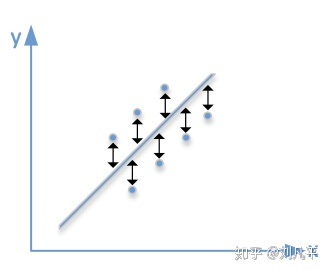最小二乘法的核心思想是通过最下化误差的平方和，试图找到最可能的函数方程 。例如在二维坐标系中存在五个数据点(10,20)、(11,23)、(12,25)、(13,27)、(14,26)，希望找出一条该五个点距离最短的直线，根据二元一次方程：y=ax+b

因此，将五个点分别带入该二元方程得到如下：

20=10a+b

23=11a+b

25=12a+b

27=13a+b

26=14a+b

由于最小二乘法是尽可能使得等号两边的方差值最小，因此 ：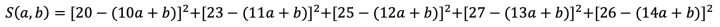因此求最小值即可通过对S(a,b)求偏导数获得，并使得一阶倒数的值为0，则：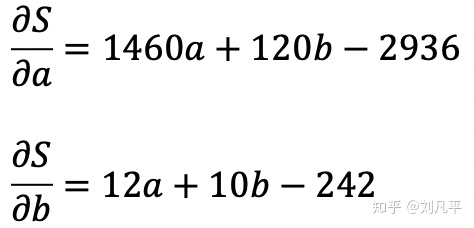即得到关于求解未知变量a、b的二元一次方程：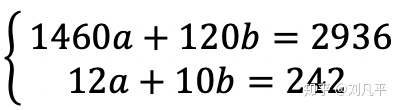通过计算上述二元一次方法即得到a=0.0243，b=24.1708。因此，在上述五个点中，通过最小二乘法得到直线方程：y=24.1708+0.0243x 是使得五个点到该直线距离最小的直线。

最小二乘法虽然看似是一个直线方程的问题，但是在实际应用中却应用非常广泛，因为它得到的方程可以视为一个函数模型，该函数模型可以对后续的工作带来极大的便利。例如在某种疾病是在两种条件下发生的，但是需要当这两种条件满足一定关系时才会促发疾病，因此医生就可以通过患病样本获得患病情况下的两种条件值，标记到一个二维坐标中，通过最小二乘法，可以将患病的两种条件通过函数表达出来，从而当有另外一个新疑似患者就医时，则可以根据二元一次方程确定是否可能患有该疾病。

上述过程均是通过线性问题的求解方式进行阐述，但是在更多的时候，需要解决的问题不是一个线性问题，它需要通过多项式拟合的方式进行处理，但是原理和求解方式均一致。虽然最小二乘法易于实现，在各行各业中都被广泛使用，但是它的计算量也比较大，当样本数据不断增加后，计算量会明显增加，在阶数更高时计算量则更为复杂。为解决更多问题，后来也基于最小二乘法衍生出了移动最小二乘法、加权最小二乘法以及偏最小二乘法等。

本文分类：传统机器学习

本文作者：刘凡平，转载或引用请声明出处，谢谢。

展开全文偏最小二乘法
• 偏最小二乘回归分析是多元线性回归分析、主成分分析和典型相关分析的有机结合,其建模原理也建立在这三种分析方法上。这里是偏最小二乘的matlab代码编写。（代码运行有问题请评论留言）
•文档
• 论文研究-偏最小二乘法在公共部门绩效多元评估中的应用.pdf, 将偏最小二乘法（PLS）应用于公共部门绩效的多元评估研究, 首先,回顾总结了PLS建模的特点及建模步骤,并就...
• errs =[]for i inrange(len(y_predict)): e= (y_predict[i]-y_test[i])**2errs.append(e)returnsum(errs)#偏最小二乘法的实现部分。 x_train, x_test, y_train, y_test = train_test_split(RON,A,test_size=0.5) #...

#-*- coding: utf-8 -*-

#看来这个程序适合的是python2版本的。来源：https://blog.csdn.net/li_huifei/article/details/78467689#这个目前也可以用了，主要还是在第60行的数据转换，，，不太清楚怎样去做装换。主要是因为数据类型不清楚，所以用了最笨的方法，不知道结果正不正确。？？？？【这个是错误的】#PLSR3经过摸索，第68行，还是因为数据结构类型不对，之后把array类型的数组转换成了list，，，这样方便在外面套一个[]，，之后运行没有问题，但是结果的正确与否，还有待验证。

#导入库的部分

importcsvfrom sklearn importpreprocessingfrom sklearn.cross_validation importtrain_test_splitfrom sklearn.decomposition importRandomizedPCAfrom sklearn.cross_decomposition import PLSRegression #偏最小二乘法的实现， 在这里是可以跳进 PLSRegression 里面的

importnumpy as npimportmathimportmatplotlib.pyplot as plt#导入数据的部分

A = np.loadtxt('A.csv',delimiter=',') #读入数据 这里的A就是y的矩阵 #读了这么多的数据？？？哪个是x，，哪个又是y呢？？？

print(A.shape)

print(RON.shape)#暂且在这里设置全局变量吧。

x_train_st_i=[]#定义全局函数的部分。

def error(y_predict,y_test): #定义计算误差平方和函数，，，传入的是估算出的值，和测试值，，这里仅仅是用来定义的，方便后面的调用。

errs =[]for i inrange(len(y_predict)):

e= (y_predict[i]-y_test[i])**2errs.append(e)returnsum(errs)#偏最小二乘法的实现部分。

x_train, x_test, y_train, y_test = train_test_split(RON,A,test_size=0.5) #划分训练集测试集，，，这个是一个库函数？？ ，，，，这里能够看出是A和RON进行建模的。

x_train_st = preprocessing.scale(x_train) #数据标准化，这个是内置函数

y_train_st = preprocessing.scale(y_train) #数据标准化,,这一句是我仿照上面的一句改写的。

n_components = 0 #这个应该是保存主成分的个数。

while n_components

n_components=n_components+1 #在第一遍的时候n_components是1，第二遍循环的时候是2，，，第n遍循环是n，，最大是x的列数，也就是特征的个数，

pls2 = PLSRegression(n_components=n_components) #计算SS （SS这个是全建模 ， PRESS是减去一个进行建模，，，，在python里建模很简单，设置好参数，调用一下函数就能建模了）

#这个不是偏最小二乘法吗？？？,,这里是循环计算主成分的个数，直到达到满意的精度。

pls2.fit(x_train_st, y_train) #fit也是一个函数，，，两个参数，第一个参数是训练集，第二个参数是目标。

y_predict0 = pls2.predict(x_train_st) #predict也是一个内置的函数，，，，这个是不是用建好的模型去做预测，，，，把参数训练集输入进去，得到的是预测的值。

SS = error(y_predict0,y_train) #这里是预测的值和真正的值之间的误差大小。

y_predict1 = [] #这是创建了一个新的变量。根据名字的意思，根据模型得到的y的预测值，实际上这个模型是留一法建立的模型。

for i in range(x_train_st.shape): #计算PRESS，，，，这个是x_train_st的行数

n_components1 = n_components #但是我不明白，为什么这里还要加1，主成分不可能是0个吧，所以就从1开始了。

x_train_st1 = np.delete(x_train_st,i,0) #这里的0是行，1是列，，这个应该是删除第i行，，，这里是标准化的数组。留一法的实现

y_train_st1 = np.delete(y_train,i,0) #这个也是删除第i行，这里都是经过标准化的（但这个x是经过标准化的，y却没有用标准化的数据）。，，这个没有用到过，是不是这里写错了？？

pls2 = PLSRegression(n_components=n_components1) #偏最小二乘法参数的设置，，，这里面一般有5个参数，，但这里只传入了主成分的个数。

#参数1：n_components:int ,(default 2) ,,要保留的主成分数量，默认为2

#参数2：scale:boolean,(default True),,是否归一化数据，默认为是

#参数3：max_iter: an integer,(default 500),,使用NIPALS时的最大迭代次数

#参数4：tol： non-negative real（default 1e-06）,,迭代截止条件

#参数5：copy： Boolean，（default True）,,

pls2.fit(x_train_st1, y_train_st1) #这里是根据前面设置好的参数建模过程，这里的建模过程是不是不太对（这里x是归一化的，y并没有用归一化的），应该都是用归一化的才行呀。？？？

#这里主要是进行了数据格式的转换，因为做预测要传进的是矩阵【格式很重要】

x_train_st_i=[] #用之前要进行清空，这个很重要。用一个参数之前要进行清空。

x_train_st_list=x_train_st[i].tolist()

x_train_st_i.append(x_train_st_list)print ('the x_train_st is',x_train_st_i) #输出一下变量，查看格式是否正确，因为下面的predict函数需要用[[1，1，1，1，1，1]] 这种格式的数据

y_predict11= pls2.predict(x_train_st_i) #预测函数，给定之前留一法没有用到的样本，进行建模，预测对应的y值。？？？？可是已经删除了不是吗？？？ ZHE这句出了一点问题？？？？就是数据格式有问题，需要在最外面在加一个[]

y_predict1.append(y_predict11)#把所有的y值组成一个数组，便于计算误差。，这个也是y的预测值，用它来算出另一个误差。

PRESS = error(y_predict1,y_train) #可能错误：https://blog.csdn.net/little_bobo/article/details/78861578

Qh = 1-float(PRESS/SS)if Qh<0.0985: #判断精度 模型达到精度要求，可以停止主成分的提取了。

plt.figure(1)

plt.scatter(y_predict0,y_train)#画了一个图，这个图是预测值，与测量值的图？？？

plt.figure(2)

plt.scatter(y_predict1,y_train)print ('the Qh is',Qh)print ('the PRESS is',PRESS)print ('the SS is',SS)break #达到了上面的精度，就可以停止while的迭代了

#这下面就没有看懂了。

print ('n_components is',n_components+1) #这里为什么要加1？？？，，，难道因为计数是从0开始的？？

SECs =[]

errors=[]

e= 100

for i in range(10): #循环测试

#print i

x_train, x_test, y_train, y_test = train_test_split( RON,A, test_size=0.5) #划分训练集与测试集，这个是一个内置的函数。

x_test_st = preprocessing.scale(x_test) #数据标准化

y_predict = pls2.predict(x_test_st) #进行预测

SECs.append(np.sqrt(error(y_predict,y_test)/(y_test.shape-1)))

errors.append(float(error(y_predict,y_test)))if SECs[-1]

y_predict_min=y_predict

y_test_min=y_testprint ('the prediced value is' ,y_predict.T) #画图，打印结果

print ('the true value is',y_test)print ('the mean error is',float(np.mean(errors)))print ("the mean SEC is",float(np.mean(SECs)))

plt.figure(3)

plt.scatter(y_predict_min,y_test_min)

展开全文• 傅立叶变换红外光谱和偏最小二乘法测定骨关节炎和健康关节软骨中胶原和蛋白聚糖的含量。研究论文
• 偏最小二乘法的几种解释

千次阅读 2020-10-17 15:05:05
经过一段时间的学习，对偏最小二乘法有了一些了解。下面主要是针对PLS1，也就是单响应变量的情况 基础准备 最小二乘法的几何意义 y=Xβ=β1x1+⋯+βnxn\mathbf{y} = X\mathbf{\beta} = \beta_1\mathbf{x_1}+\dots+\...

经过一段时间的学习，对偏最小二乘法有了一些了解，这里做一个总结。下面主要是针对PLS1，也就是单响应变量的情况

基础准备

最小二乘法的几何意义

y = X β = β 1 x 1 + ⋯ + β n x n (1) \mathbf{y} = X\mathbf{\beta} = \beta_1\mathbf{x_1}+\dots+\beta_n\mathbf{x_n} \tag{1}找到一个 X X 的列的线性组合，使得这个线性组合最多的表达 y y 中的信息。 由上图可知， y = y p + y e y = y_p+y_e .当 y e y_e 正交于 X X 列空间时，达到最小，此时， y p y_p y y 的夹角最小，即相关性最大。普通最小二乘法（OLS）的解如下
β ^ = ( X T X ) − 1 X T y \hat{\beta}=(X^TX)^{-1}X^Ty

典型相关分析

典型相关分析是从两组数据 X X Y Y 中，找到两个相应的组合，使得两者相关性最大。其目标如下
a r g   m a x w , c [ ( X w ) T ( Y c ) ] 2 ∣ ∣ ( X w ) ∣ ∣ 2 ∣ ∣ ( Y c ) ∣ ∣ 2 ⇔ a r g   m a x w , c   c o s ( X w , Y c ) 2 (2) arg \ \underset{w,c}{max} \frac{[(Xw)^T(Yc)]^2}{||(Xw)||^2||(Yc)||^2} \Leftrightarrow arg \ \underset{w,c}{max} \ cos(Xw,Yc)^2 \tag{2}

G c c a = [ ( X w ) T ( Y c ) ] 2 ∣ ∣ ( X w ) ∣ ∣ 2 ∣ ∣ ( Y c ) ∣ ∣ 2   ∈ [ 0 , 1 ] G_{cca} = \frac{[(Xw)^T(Yc)]^2}{||(Xw)||^2||(Yc)||^2} \ \in [0,1]

在单响应变量的情况下，则有 X w Xw y y 的相关性最大，则可以得到

w ∝ ( X T X ) − 1 X T y w \propto (X^TX)^{-1}X^Ty

主成分分析

PCA的思想是找到数据 X X 中代表最大方差方向的权值，通过减秩消除矩阵中的无关信息这个方向的确定很容易，即 X T X X^TX 的最大特征向量
w ^ = a r g   m a x w ^   w T X T X w = a r g   m a x w ^ w T X T X w [ ρ ( X T X ) ] 2 s . t .   ∣ ∣ w T w ∣ ∣ = 1 (3) \hat{w} = arg \ \underset{\hat{w}}{max} \ w^TX^TXw =arg \ \underset{\hat{w}}{max} \tfrac{w^TX^TXw}{[\rho(X^TX)]^2} \tag{3}\\ s.t. \ ||w^T w||=1
G p c r = w T X T X w [ p ( X T X ) ] 2 G_{pcr} = \tfrac{w^TX^TXw}{[p(X^TX)]^2} , G p c r ∈ [ 0 , 1 ] G_{pcr} \in [0,1]
对比OLS和PCA可以发现，前者的目标是使得 G c c a G_{cca} 最大化,后者是使 G p c r G_{pcr} 最大化。在实际应用中，两个目标往往难以同时达到最大。

第一种解释

P L S 可 以 看 作 是 C C A 和 P C A 的 一 个 折 中 。 \color{red}{PLS可以看作是CCA和PCA的一个折中。}
PLS是一种数据减秩的方法，跟PCA类似，是用原数据的部分数据（成分）代替原始数据。构造成分的方法和CCA，PCA不同之处在于，PLS是两者的一个平衡点，由下面的目标式可以清楚得看到。
w ^ = a r g   m a x w ^   ( y T X w ) 2 = a r g   m a x w ^ ( ( y T X w ) 2 y T y w T X T X w ) ( ( w T X T X w ) [ ρ ( X T X ) ] 2 ) ( y t y ) = a r g   m a x w ^   G c c a G p c r s . t .   ∣ ∣ w T w ∣ ∣ = 1 (4) \hat{w} = arg \ \underset{\hat{w}}{max} \ (y^TXw)^2 =arg \ \underset{\hat{w}}{max} (\tfrac{ (y^TXw)^2}{y^Tyw^TX^TXw})(\tfrac{ (w^TX^TXw)}{[\rho(X^TX)]^2} )(y^ty) =arg \ \underset{\hat{w}}{max} \ G_{cca}G_{pcr} \tag{4}\\ \\s.t. \ ||w^T w||=1
更直观一些看下图，假设 ∣ ∣ w ∣ ∣ = 1 ||w||=1 ，对于所有的可能的点 X w Xw ，构成了如下的的超椭圆空间。CCA,PCR，PLS在成分或者说得分向量的构造方式上存在以下的几何关系。具体可以看参考文献部分

第二种解释

P L S 是 一 种 共 轭 梯 度 法 \color{green}{PLS是一种共轭梯度法}上图中，左边是最速下降法的优化路径，右图是共轭梯度下降法的优化路径。可见，共轭梯度法的效率要远高于最速下降法的速率。直观得看，最速下降法的缺点在走回头路，导致收敛速度缓慢，共轭梯度法的特点保证了其不走回头路，所以，收敛的速度是有保障的。所谓共轭，就是权值方向满足
w i T X T X w j = 0 , i ≠ j (5) w_i^TX^TXw_j=0,i \neq j \tag{5}
这个的证明可以看前面的文章。无论是NIPALS（基于残差），SIMPLS（基于载荷矩阵正交投影）这一点都是可以满足的。

从几何的角度来看，构造共轭正交的权值，是为了保证得分向量 t = X w t=Xw 的正交性，因为得分矩阵 T T 最终代替X， 响应变量 y y 最终通过将自身投影到 T T 的空间中，获得 y y T T 的回归关系。如下图所示， t t 是两两正交的，新增的 t i t_i 不影响原来 y y 在其他 t j , j < i t_j,j<i 上的投影, y y 因此一定是收敛的。第三种解释

P L S 是 一 种 基 于 K r y l o v 空 间 降 维 打 击 的 方 法 。 \color{blue}{PLS是一种基于Krylov空间降维打击的方法。}

尽管Krylov空间和共轭梯度法是密不可分的，这里还是单独从这个角度谈谈。
Krylov subspaces 子空间的形式如下
K q = s p a n ( s , S s , S 2 , … , S q − 1 s ) (6) K_q = span(s,Ss,S^2,\dots,S^{q-1}s) \tag{6}
KryLov空间常用于求解大型矩阵的逆
A x = b ⇒ x = A − 1 b A − 1 b ≈ β 0 b + β 1 A b + β 2 A 2 b + ⋯ + β r A r b = ∑ i = 0 r β i A i b A i b   c a n   b e   o b t a i n e d   b y   J a c o b i a n   F r e e   N e w t o n   K r y l o v A ( ∑ i = 0 r β i A i b ) = b ⇒ A − 1 b (7) Ax = b \Rightarrow x = A^{-1}b\\ A^{-1}b \approx \beta_0b+\beta_1Ab+\beta_2A^2b+\dots+\beta_rA^rb=\sum_{i=0}^{r}\beta_iA^ib \tag{7}\\ A^ib\ can\ be\ obtained\ by \ Jacobian\ Free\ Newton\ Krylov\\ A(\sum_{i=0}^{r}\beta_iA^ib) = b\Rightarrow A^{-1}b
和PLS的关系

L e t   s = X T y , S = X T X f o r   a l l   w i ∗ , i ∈ ( 1 , r )   s p a n   s p a c e   K r K r = s p a n ( s , S s , S 2 s , … , S r − 1 s ) t i = X w i ∗ y ^ = X β ^ = λ 1 t 1 + ⋯ + λ r t r ∗ ⇒ β ^ P L S = λ 1 w 1 ∗ + ⋯ + λ r w r ∗ = ∑ i = 0 r − 1 β i S i s (8) Let \ s= X^Ty ,S = X^TX \\ for\ all \ w_i^*,i \in (1,r)\ span\ space\ K_r\\ K_r = span(s,Ss,S^2s,\dots,S^{r-1}s) \\ t_i = Xw_i^*\\ \\\hat{y}=X \hat{\beta} =\lambda_1t_1+\dots+\lambda_rt_r^* \Rightarrow\\\tag{8} \hat{\beta}_{PLS} = \lambda_1w^*_1+\dots+\lambda_rw_r^* =\sum_{i=0}^{r-1}\beta_iS^is

只要 r r 足够大， β ^ P L S → β ^ O L S \hat{\beta}_{PLS} \rightarrow \hat{\beta}_{OLS}

第四种解释

最 大 化 信 噪 比 方 向 \color{#fbbc05}{最大化信噪比方向}
Maximinze Signal-To-Noise Ratio(SNR)体现了PLS在权值w上的选取意义
PLS的解是是有偏估量，本质上是以无偏估量 β O L S \beta_{OLS} 解作为信号，以最大化信噪比的方向去提取 β O L S \beta_{OLS} 中的信息，构造近似的解。
按照有偏估量的计算，可以得到如下方程
a r g   m a x w q   ∣ w T β ^ O L S ∣ σ w T ( X T X ) − 1 w ⇔ a r g   m a x w q   c o s ( w , β ^ O L S ) 2 w T ( X T X ) w ,   s . t .   w q ⊥ ( w 1 , w 2 , … , w q − 1 ) (10) arg \ \underset{w_q }{max} \ \frac{|w^T\hat{\beta}_{OLS}|}{\sigma\sqrt{w^T(X^TX)^{-1}w}}\Leftrightarrow arg \ \underset{w_q }{max} \ cos(w,\hat{\beta}_{OLS})^2w^T(X^TX)w ,\tag{10}\\ \ s.t. \ w_q \perp (w_1,w_2,\dots,w_{q-1})
看上面左边的公式，分子部分代表了相关性，即信息最大，分母部分代表了噪声的估计量。两者结合在一起就是信噪比，这和PLS的目标是一致的。
对上式重写，可以转换为如下的形式
a r g   m a x w q   ∣ w q T β ^ O L S ∣ , s . t .   w q ⊥ ( w 1 , w 2 , … , w q − 1 ) ,   w q T S − 1 w q = 1 arg \ \underset{w_q }{max} \ |w_q^T\hat{\beta}_{OLS}| , s.t. \ w_q \perp (w_1,w_2,\dots,w_{q-1}), \ w_q^TS^{-1}w_q=1

λ q w q = − s − ∑ i = 1 q − 1 λ i ∗ S ∗ w i ⇒ β ^ P L S = λ 1 w 1 + ⋯ + λ r w r \lambda_qw_q = -s - \sum_{i=1 }^{q-1}\lambda_i*S*w_i \Rightarrow \hat{\beta}_{PLS} = \lambda_1w_1+\dots+\lambda_rw_r

总结

偏最小二乘当然还有许多其他的解释，这里不再一一介绍。一直以来，偏最小二乘法总是偏向于直觉，而缺乏坚实的理论而受到诟病，特别是统计学界。另外，目前的文献资料显示，偏最小二乘法的解绝并非统计学意义上的最优解，这一点已有诸多文献讨论并且做出相关证明。但由于其在小样本，高维共线的领域里出色效果，在化学计量，经济计量等受到广泛的应用。这里主要是将自己对偏最小二乘法的理解总结一下，以后大概不会再写普通的偏最小二乘法，更多地是讨论一下改进的偏最小二乘法，如稀疏，鲁棒等版本的偏最小二乘模型。

参考文献

Optimizing a vector of shrinkage factors for continuum regression

展开全文偏最小二乘法
• 偏最小二乘法

2013-08-13 10:23:53
基于偏最小二乘回归分析综述，讲述偏最小二乘法的求解方法偏最小二乘
• 化学计量学方法中常用的一种建模方法，可以通过通过最小化误差的平方和找到一组数据的最佳函数匹配。 用最简的方法求得一些绝对不可知的真值，而令误差平方之和为最小。 很多其他的优化问题也可通过最小化能量或最大...
• 偏最小二乘法 Partial Least Squares

万次阅读 多人点赞 2019-06-24 00:15:58
本文前部分摘自：偏最小二乘法回归（Partial Least Squares Regression），后半部分原创。 诸如基因组学、转录组学、蛋白组学及代谢组学等高通量数据分析，由于自变量数目大于病例数（未知数大于方程个数），无法...

本文前部分摘自：偏最小二乘法回归（Partial Least Squares Regression），后半部分原创。

诸如基因组学、转录组学、蛋白组学及代谢组学等高通量数据分析，由于自变量数目大于病例数（未知数大于方程个数），无法直接使用传统的统计分析模型。比如，线性回归的窘境：如果样例数m相比特征数n少（m<n）或者特征间线性相关时，由于（n*n矩阵）的秩小于特征个数（即不可逆）。因此最小二乘法就会失效。遇到这种情况，需要先降维处理。有监督的降维方法，除了常用的LASSO，还有一种叫PLS（偏最小二乘法 Partial Least Squares，或称PLSR 偏最小二乘法回归）。PLSR感觉已经把成分分析和回归发挥到极致了。本文将介绍PLS的原理和应用。

主成分分析 PCA

先回顾下PCA。令X表示样本，含有m个样例，每个样例特征维度为n，。假设我们已经做了每个特征均值为0处理。

如果X的秩小于n，那么X的协方差矩阵 的秩小于n，因此直接使用线性回归的话不能使用最小二乘法来求解出唯一的，我们想使用PCA来使得可逆，这样就可以用最小二乘法来进行回归了，这样的回归称为主元回归（PCR）。

PCA的一种表示形式：T=XP

其中X是样本矩阵，P是X的协方差矩阵的特征向量（当然是按照特征值排序后选取的前r个特征向量），T是X在由P形成的新的正交子空间上的投影（也是样本X降维后的新矩阵）。

PCA的另一种表示：

（假设X秩为n）

这个公式其实和上面的表示方式T=XP没什么区别。

（当然我们认为P是n*n的，因此

如果P是n*r的，也就是舍弃了特征值较小的特征向量，那么上面的加法式子就变成了

这里的E是残差矩阵。其实这个式子有着很强的几何意义， 大特征值对应的归一化后的特征向量，就是X在上的投影。就是X先投影到上，还以原始坐标系得到的X’。下面这个图可以帮助理解：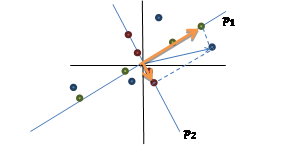黑色线条表示原始坐标系，蓝色的点是原始的4个2维的样本点，做完PCA后，得到两个正交的特征向量坐标。绿色点是样本点在上的投影（具有最大方差），红色点是在上的投影。的每个分量是绿色点在上的截距，是红色点在上的截距。中的每个分量都可以看做是方向为，截距为相应分量大小的向量，如那个上的橘色箭头。就得到了X在的所有投影向量，由于正交，因此就相当于每个点的橘色箭头的加和，可想而知，得到了原始样本点。

PLSR思想及步骤

我们还需要回味一下CCA来引出PLSR。在CCA中，我们将X和Y分别投影到直线得到u和v，然后计算u和v的Pearson系数（也就是Corr(u,v)），认为相关度越大越好。形式化表示：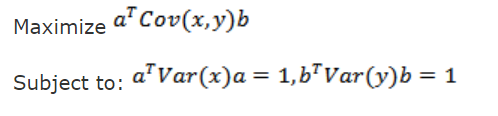其中a和b就是要求的投影方向。

想想CCA的缺点：对特征的处理方式比较粗糙，用的是线性回归来表示u和x的关系，u也是x在某条线上的投影，因此会存在线性回归的一些缺点。我们想把PCA的成分提取技术引入CCA，使得u和v尽可能携带样本的最主要信息。还有一个更重要的问题，CCA是寻找X和Y投影后u和v的关系，显然不能通过该关系来还原出X和Y，也就是找不到X到Y的直接映射。这也是使用CCA预测时大多配上KNN的原因。

而PLSR更加聪明，同时兼顾PCA和CCA，并且解决了X和Y的映射问题。看PCA Revisited的那张图，假设对于CCA，X的投影直线是，那么CCA只考虑了X的绿色点与Y在某条直线上投影结果的相关性，丢弃了X和Y在其他维度上的信息，因此不存在X和Y的映射。而PLSR会在CCA的基础上再做一步，由于原始蓝色点可以认为是绿色点和红色点的叠加，因此先使用X的绿色点对Y做回归（ ，样子有点怪，两边都乘以就明白了，这里的Y类似于线性回归里的类似 ），然后用X的红色点对Y的剩余部分F做回归（ 得到 ）。这样Y就是两部分回归的叠加。当新来一个x时，投影一下得到其绿色点和红色点，然后通过r就可以还原出Y，实现了X到Y的映射。当然这只是几何上的思想描述，跟下面的细节有些出入。

下面正式介绍PLSR：

1） 设X和Y都已经过标准化（包括减均值、除标准差等）。

2） 设X的第一个主成分为，Y的第一个主成分为，两者都经过了单位化。（这里的主成分并不是通过PCA得出的主成分）

3） ，这一步看起来和CCA是一样的，但是这里的p和q都有主成分的性质，因此有下面4）和5）的期望条件。

4） ，即在主成分上的投影，我们期望是方差最大化。

5） ，这个跟CCA的思路一致。

6） 综合4）和5），得到优化目标 ，即协方差最大化。

形式化一点：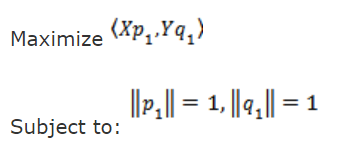先看该优化问题的求解吧：引入拉格朗日乘子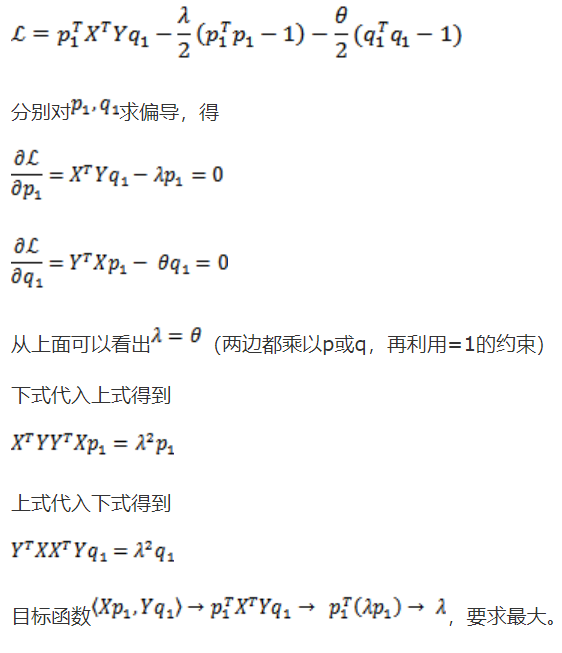因此就是对称阵的最大特征值对应的单位特征向量，就是最大特征值对应的单位特征向量。

可见是投影方差最大和两者相关性最大上的权衡，而CCA只是相关性上最大化。

求得了，即可得到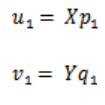利用我们在PCA Revisited里面的第二种表达形式，我们可以继续做下去，建立回归方程：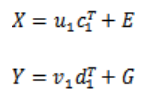这里的c和d不同于p和q，但是它们之间有一定联系，待会证明。E和G是残差矩阵。

我们进行PLSR的下面几个步骤：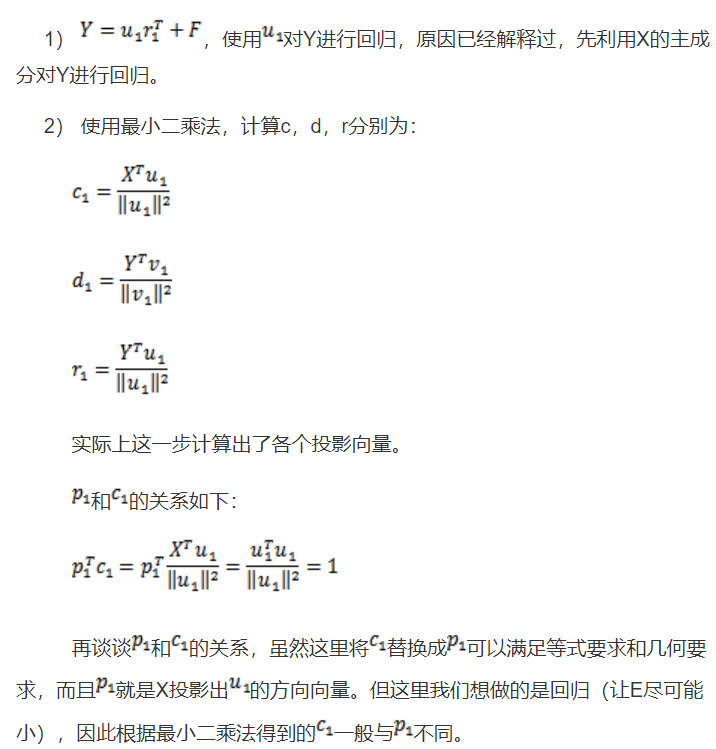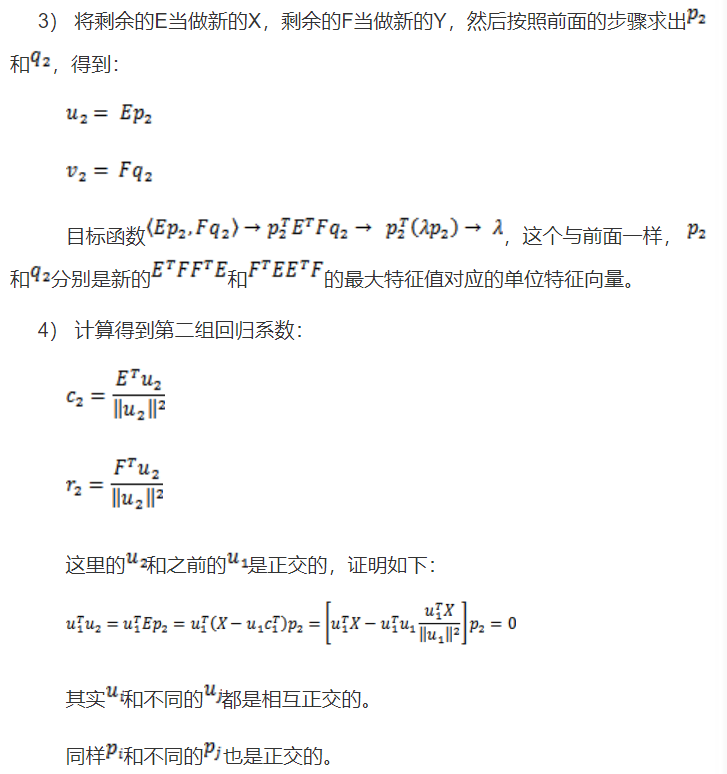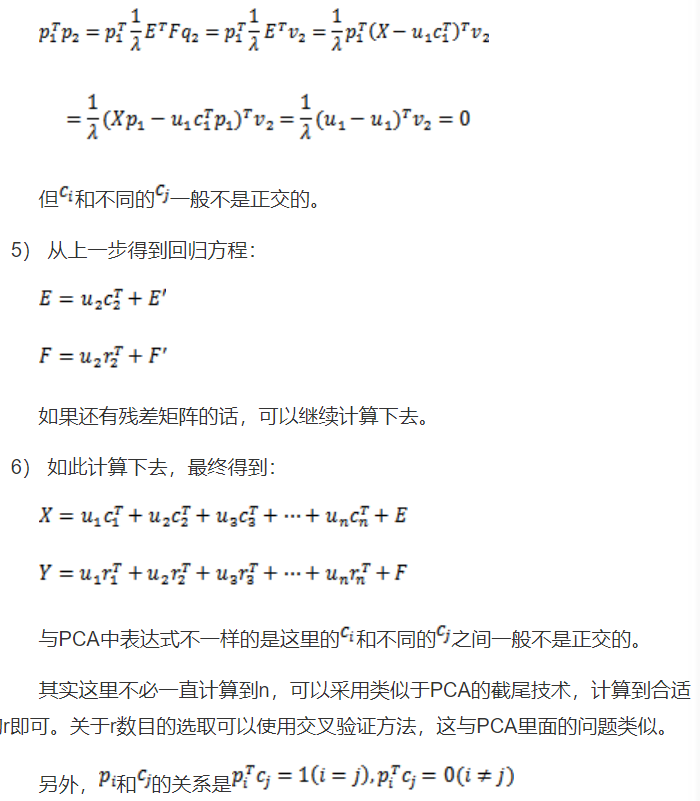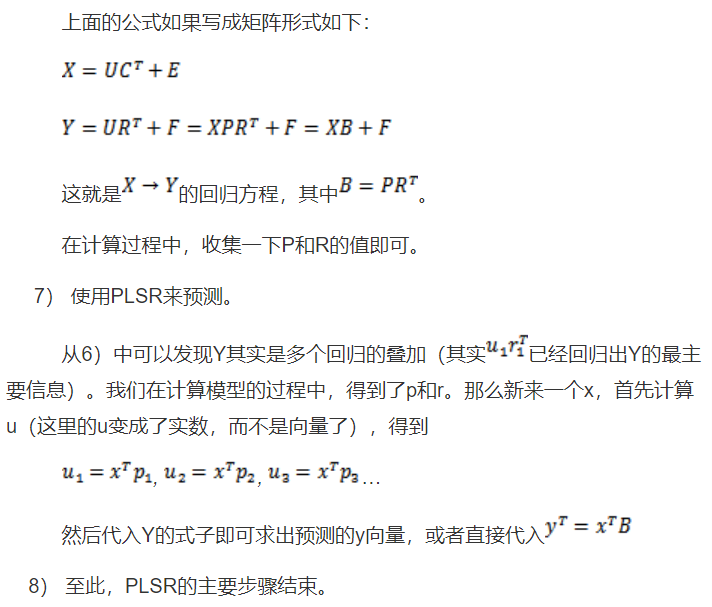由上述步骤可知，虽然PLS多次迭代映射分解，但是最终组合起来，仍然是一个Y与X的线性回归Y=XB+F！将每次迭代过程中的映射变换的参数进行线性运算可以得到最终模型的参数B和F。

上述步骤中，矩阵C即为载荷矩阵（即通过可计算出每个样本在第 i 个成分的得分）。

PLS结果的解读

（本部分查阅资料后总结）

通过PLS分析，可以得到PLS-VIP值和PLS-BETA值，可用于筛选变量（PLS-VIP最常使用）。

PLS-VIP

PLS-VIP指的是Variable Importance in Projection，最先在 3D QSAR in Drug Design: Theory Methods and Applications 这本书中提出（可惜这本书很贵，看不到相应的描述）。第 j 个变量的VIP值计算方法如下：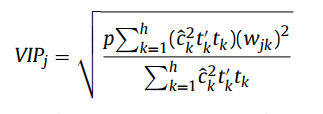其中，p表示最初总共有p个变量参与分析；h表示最终总共进行了h次迭代计算（总共获得了h个维度）；表示第k次迭代时（第k个维度），变量 j 进行映射时所采用的权重（即协方差矩阵中的系数），反应的是变量 j 对第k次映射结果的解释程度；表示第k次映射的结果 对  的解释程度（ 注：是自变量X第k次的映射结果，是因变量Y在第k次迭代时被解释的部分 ）。

由于所有变量的VIP值的平方和等于1，所以往往将1作为VIP判定的界值：一般认为VIP小于1的变量对模型解释程度很低。

PLS-BETA

PLS-BETA值的是PLS最终回归模型中各个变量的系数。上一部分的推导结果可知，本质上最终的模型仍然是Y与X的线性回归Y=XB+F，这里的B即为系数向量。线性回归模型的系数越大往往预示着这个变量越重要（前提是各个变量已经标准化处理）。PLS-BETA 在许多文献里写作 Cov(t, Xi)，即得分矩阵与自变量矩阵的的协方差。

Correlation Coefficient

Correlation Coefficient 指的是样品得分与变量之间的相关性（即预测的Y与每个变量之间的相关性r），简称Corr.Coeffs，这其实是对PLS-BETA的补充。相关性越好，则说明该变量越重要。这个相关性还可以计算显著性p值（同理，PLS-BETA也可以计算p值），相关性r的界值没有规定，一般根据其对应的p值取0.05作为界值。

Corr.Coeffs在许多文献里也写作 Corr(t, Xi)，即得分矩阵与自变量矩阵的的相关系数。根据协方差与相关系数的数学关系可知：Corr(t, Xi) = Cov(t, Xi) / (St * Sxi)。

V-Plot

同时参照VIPCorr.Coeffs筛选变量，是常用的策略。由于大多数情况下，VIP值和Corr.Coeffs值相关性较好，且VIP恒正而Corr.Coeffs可正可负，因此可视化的图类似于V形，被称为V-Plot。

S-Plot

同时参照PLS-BETACorr.Coeffs筛选变量，也是常用的策略。由于PLS-BETACorr.Coeffs同正同负，因此可视化的图中所有的点全部分布在第一和第三象限，类似于S形，被称为S-Plot。

S-Plot 详细的解读，可以参考原始论文：Visualization of GC/TOF-MS-Based Metabolomics Data for Identification of Biochemically Interesting Compounds Using OPLS Class Models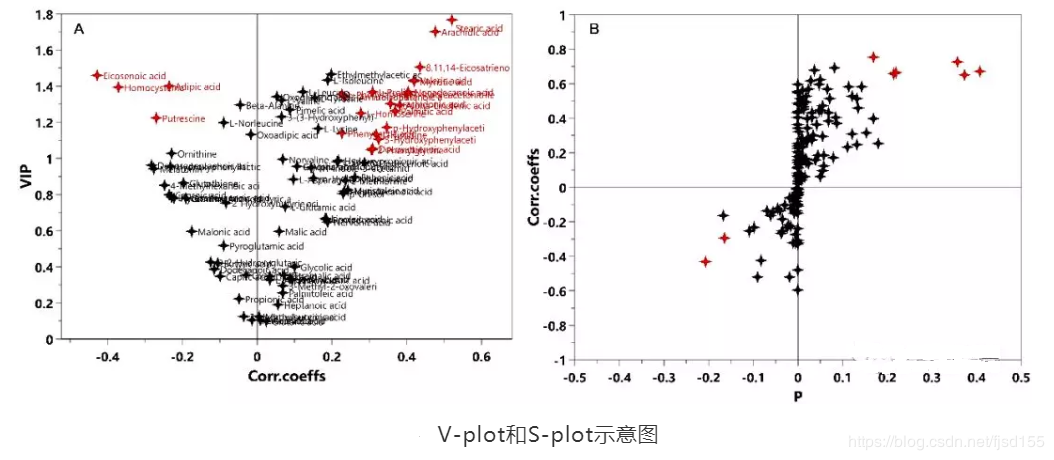原始论文部分截图如下：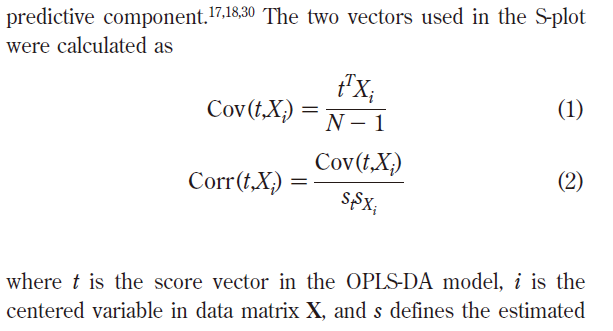PCA的二维 score plot 的含义是 以这两个主成分作为自变量绘制二维坐标系，各个样本的坐标值是多少。可以看出 score plot是针对样本而言。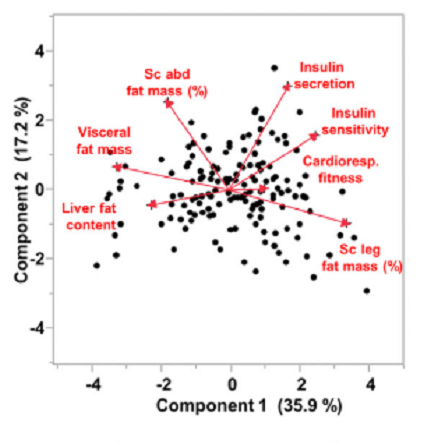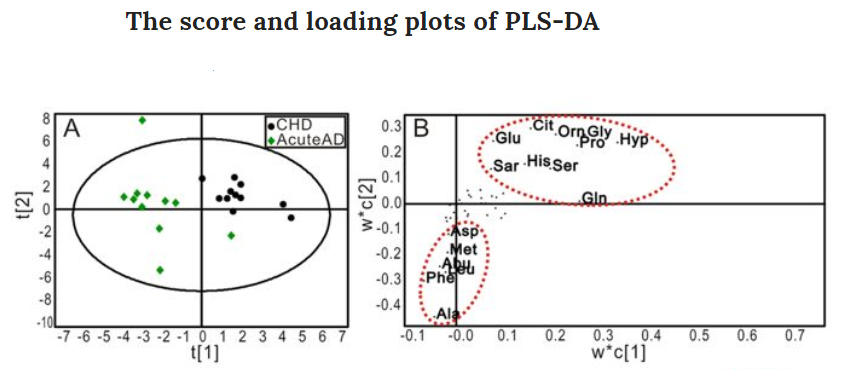这部分理解还可以参考：

成分分析中biplot函数

样本得分图和自变量的载荷图如何出现在一张图上

PLS模型评价

（本部分查阅资料后总结）

拟合效果

评价PLS-DA 模型拟合效果使用R2X、R2Y和Q2Y这三个指标，这些指标越接近1 表示PLS-DA 模型拟合数据效果越好。其中，R2X 和R2Y 分别表示PLSDA分类模型所能够解释X 和Y 矩阵信息的百分比，Q2Y 则为通过交叉验证计算得出，用以评价PLS-DA模型的预测能力，Q2Y 越大代表模型预测效果较好。

Q2和R2从计算上来看其实一模一样，只是采用的样本不一样，公式都是 1 - RSS/TSS（Q2中一般把RSS改写为PRESS，即 predicted residual error sum of squares）。R2是对 参与拟合的样本 计算残差，因此体现的是“拟合能力”；Q2是对 交叉验证中未参与拟合的样本 计算残差，因此体现的是“预测能力”。由于R2进行计算时，拟合后残差肯定不会变大，所以R2的取值在0-1之间；Q2中残差可能会比初始状态的残差更大，因此Q2甚至有可能为负数。另外，对于每个自变量都可以计算对应的R2和Q2。

从《Multi- and Megavariate Data Analysis Basic Principles and Applications》书中截取的公式如下（这本书比较权威）：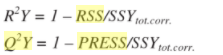完整的原文如下：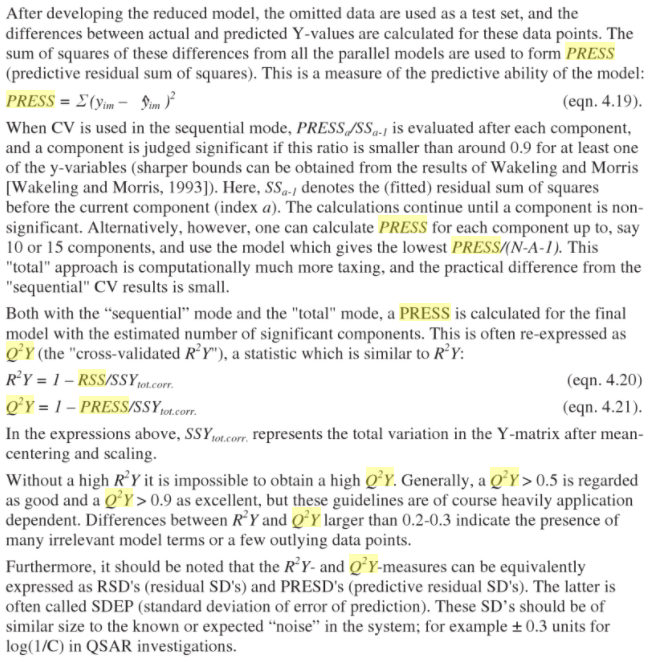随着加入模型的变量增加（模型变复杂），R2会增加或进入平台期，但Q2可能会下降（Q2下降说明过拟合了），回想一下测试集和训练集的学习曲线可知。我们需要找一个平衡，其实也就是“方差variance”和“偏差bias”的平衡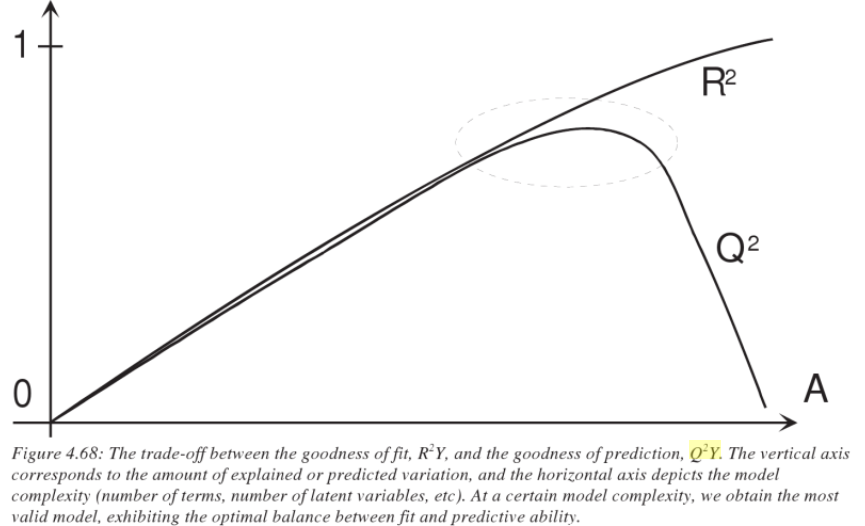PCA分析中R2X >0.4为好（PCA中只有R2X，即最终纳入的几个成分累计对原始变量的还原程度 [或者说解释程度] ）；PLS-DA 和 OPLS-DA分析中，R2X 这个参数不重要了，主要是R2Y 和Q2，这两个值>0.5 为好，越接近1越好。我们经常看到PLS-DA中有个Q2(cum)，是指建模后模型的预测能力（即最终得到线性模型Y=XB+F的预测能力），以大于0.5为宜，越接近1越好，cum 表示累积的意思，在公式中涉及到单自变量Q2的累乘运算，但其实就是模型整体的Q2。

是否过拟合

可靠的解读，需要进行permutation test（置换检验）判断模型是否过拟合。一般需检验模型的Q2值和R2值。对于Q2，要求置换检验结果的在y轴上的截距小于0，方可认为模型没有过拟合。这种标准相对严格，可能是考虑到：置换检验中标签打乱的比例和拟合/预测能力成正比例函数时（过原点坐标），过拟合情况正好消失（仅保留了该有的拟合能力），若稍微存在一定过拟合，则截距会在原点之上。但是，也有另一种宽松的标准，只要能推测出“只有极少数（5%）置换模拟的Q2值比当前Q2值大”，则认为没有过拟合（ropls包中采用的是这种宽松的方式，给出置换检验的p值进行判断）。

关于这个问题，笔者后来在《Multi- and Megavariate Data Analysis Basic Principles and Applications》书中得到了说法：根据经验来看，R2截距应小于0.3，Q2截距应小于0.05。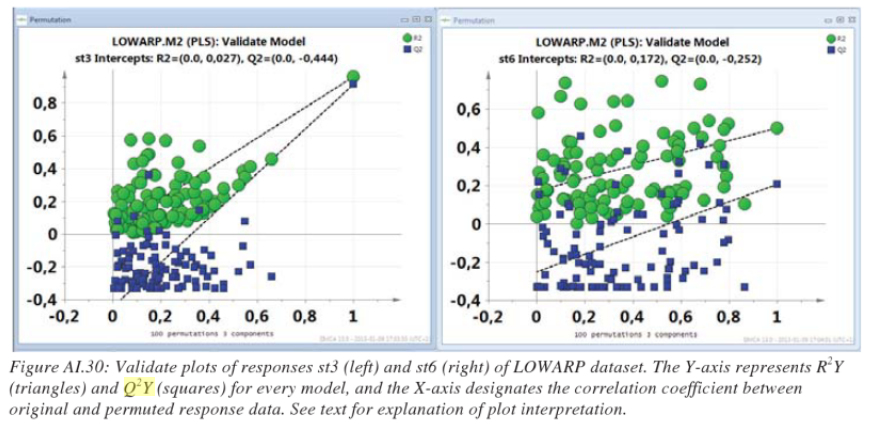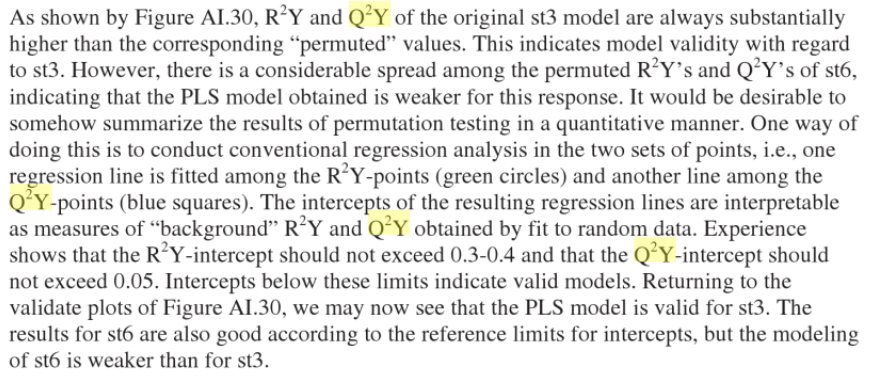置换检验的基本原理：将真实分类结果（标签）屏蔽，重新随机赋予分类结果（标签），再进行建模。如果真实建模的Q2和随机标签建模的Q2接近，则说明模型过拟合。具体原理请参考其他资料。置换检验可视化的图，横坐标表示的是置换后的标签与真实标签的相似性（有多少比例的样本未打乱重新赋予标签）。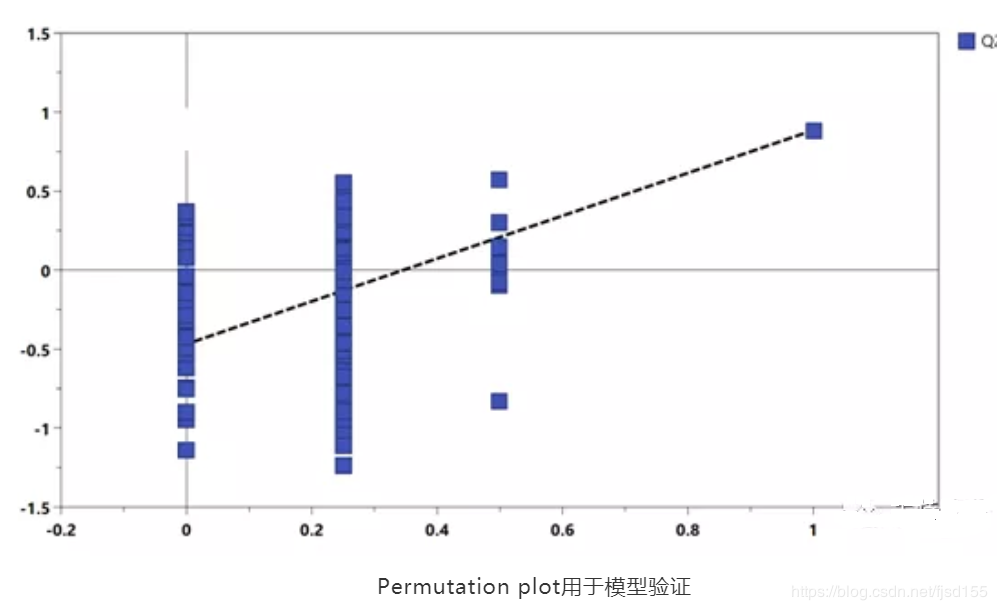PLS-DA

PLS本质上是线性回归，若遇到分类任务，需要将因变量进行dummy处理（哑变量化），此时便称为PLS-DA。为了与PLS-DA区分，普通的PLS又称为PLSR。

笔者有个疑惑，对于二分类问题，直接使用PLSR进行处理是否合理？之前在SVM中也遇到过相似的疑惑：普通的二分类问题，用SVR处理是否合理？笔者之前的一些任务中，发现二分类中可以使用SVR替代SVM。但是解决这些疑惑还需要重新解读PLS-DA及SVR的原理，以及进行一些模拟数据的测试。

根据笔者的经验，将连续性因变量问题转为分类问题，可以仿照 Logistic，在原先回归的基础上再加一个连接函数（如Logistic 中的连接函数为logit 函数 [ 其反函数是 sigmoid 函数 ] ），将问题转为广义线性回归的任务。PLS 与 PLS-DA 之间的转换，为什么不采用这种技巧呢？

PLS 常用R工具包：ropls、pls、plsdepot 及 mixOmics；常用的python工具包：sklearn.cross_decomposition 中的 PLSRegression。

ropls包使用比较简单，可以跟着提供的案例进行学习。

pls 包是最基础的工具包，里面有封装了各种求解 PLS 的算法，其中的 CPPLS 算法可以解决多个因变量的问题及分类问题（分类问题常常使用哑变量法，被转换为多因变量问题）。但是 pls 包不能直接计算 vip 值，需要自己手动编写计算程序。

plsdepot 包也是值得推荐的工具包，里面同样封装了很多PLS 相关的算法。其中，plsreg1 可以处理普通的PLS任务，而plsreg2可以处理多个因变量的问题（也就可以处理分类问题）。另外，pslreg2 可以计算 vip 值，并且还计算了Q2等结果。

mixOmics 是另一个不错的工具，可以直接计算 PLS 和 PLS-DA等问题。mixOmics 中的vip方法可以计算 vip 值

一个题外话——哑变量技巧

对于存在分类变量的问题，我们第一步就是对变量进行dummy处理。对于自变量的哑变量化与因变量的哑变量化，有一些细微的区别。

对于类别数为 k 的分类自变量，我们会设置（k-1）个哑变量：。哑变量的编码规则为：对于参照类别（又称类别0，可以人为定义一个类别作为计算的参照值，等价于连续性变量中的0值），我们将各个哑变量都赋值为0，即： ；对于其他类别，我们为每个类别选择一个哑变量，并将相应的哑变量赋值为1 而其他哑变量赋值 0，比如类别1可以这样赋值： 。这么处理是为了在结果解读时，能够为每个哑变量找到“参照值”。

对于类别数为 k 的分类因变量，我们会设置 k 个哑变量： 。为每个类别选择一个哑变量，并将相应的哑变量赋值为1 而其他哑变量赋值为 0。这种处理技巧很常见，理解也比较简单。比如 Logistic 的 softmax 函数也是采用哑变量的形式处理多分类问题。

OPLS及OPLS-DA

（本部分出处忘记了）

OPLS-DA是PLS-DA的扩展，即首先使用正交信号校正技术，将X矩阵信息分解成与Y相关和不相关的两类信息，然后过滤掉与分类无关的信息，相关的信息主要集中在第一个预测成分。Trygg et al. (2002)认为该方法可以在不降低模型预测能力的前提下，有效减少模型的复杂性和增强模型的解释能力。与PLS-DA模型相同，可以用R2X、R2Y、Q2和OPLS-DA得分图来评价模型的分类效果。目前一般认为，在二分类问题中，OPLS-DA可以作为首选分析方法（代谢组学中更多人使用OPLS，而不是PLS）。

PLS的一些思考

PLS与LASSO的对比

（本部分原创）

PLS和LASSO，从本质上来讲，都是线性回归模型！只是说，这二者在进行回归建模时，顺便进行了特征降维。

在完成降维后，二者重新构建模型，得到的其实就是一个简单的线性回归模型。而在变量选择（降维）的过程中，两者采用的策略不同，PLS采用的是成分分解分析的思路，而LASSO采用的是惩罚约束策略。

至于具体哪种方法更好，很难说。在实际数据分析时，两种方法都可以进行尝试，最后选择效果更好的方法即可。

在应对共线性（collinearity）问题上，两者的表现如何呢？由于LASSO的原则是使模型尽可能简单，也就会尽可能消除共线性；但是PLS关注的是各个变量对Y的解释，会保留共线性的变量。2005年有篇论文（Performance of some variable selection methods when multicollinearity is present）对此进行了验证，结论里提到“The PLS-VIP method performed excellently in identifying relevant predictors and outperformed the other methods. It was also found that a model with good fitness performance may not guarantee good variable selection performance. Thus, for the purpose of selecting relevant process variables, process engineers must be careful when using model performance such as RMSE, R-squares, etc.”  该论文作者提出，在选择变量时，尽量避免使用R2等评价指标，而应该直接使用上述结果解读部分的方法。

PLS与GBDT的对比

朴素的思想：PLS和GBDT的拟合策略背后，都蕴含着一种由简入繁的思想，先拟合大致轮廓，再逐步拟合细节。

贪心的思想：局部最优化，PLS与GBDT都是考虑当前的最优拟合决策，这是典型的贪心拟合策略。贪心的解未必是全局最优解。

PLS与GBDT的不同：PLS本质上还是线性模型，GBDT可以是非线性的。PLS的生物学解释性较强，GBTD建立的模型未必能很好地解释（决策树类的模型关注决策条件的信息熵，往往会忽视变量的生物学解释；线性模型可以保留生物学线性关系，但无法考虑到潜在的交互作用 [除非添加交互项] ）。

参考资料

偏最小二乘法回归（Partial Least Squares Regression）

N.L. Afanador, et al. Use of the bootstrap and permutation methods for a more robust variable importance in the projection metric for partial least squares regression. Analytica Chimica Acta. 2013

Il-Gyo Chong, et al. Performance of some variable selection methods when multicollinearity is present. Chemometrics and Intelligent Laboratory Systems. 2005

代谢组学数据分析的统计学方法综述

展开全文偏最小二乘法 PLSR
•研究论文
• 偏最小二乘法代码偏最小二乘法 (PLS)、基于核的潜在结构正交投影 (K-OPLS) 和基于 NIPALS 的 OPLS 基于Yi Cao实现的PLS回归算法： 基于 的 K-OPLS 回归算法。 基于 R 包的 OPLS 实现，使用 NIPALS 分解循环。 安装 \$...
• pls偏最小二乘法的matlab实现，网上下载的，都打包到一起了，慢慢看吧。我从中找到了自己想要的，希望对你有帮助！
• 可以对光谱数据进行预处理，除了偏最小二乘法之外，也有一些其他方法...